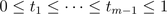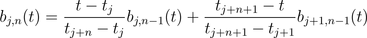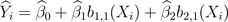Want to share your content on R-bloggers? click here if you have a blog, or here if you don't.

Splines in regression is something which looks like a black box (or maybe like some dishes you get when you travel away from home: it tastes good, but you don’t what’s inside… even if you might have some clues, you never know for sure*).With splines, it is the same: there are knots, then we consider polynomial interpolations on parts between knots, and we make sure that there is no discontinuity (on the prediction, but on the derivative as well).
That sounds nice, but when you look at the output of the regression… you got figures, but you barely see how to interpret them… So let us have a look at the box, and I mean what is inside that box…So, consider the following dataset, with the following spline regression,
> library(splines)
> K=c(14,20)
> plot(cars)
> reg=lm(dist~bs(speed,knots=c(K),
+ degree=2),data=cars)
> u=seq(4,25,by=.1)
> B=data.frame(speed=u)
> Y=predict(reg,newdata=B)
> lines(u,Y,lwd=2,col=”red”)
I.e. we have the following (nice) picture,But if we look at the output of the regression, we get this
> summary(reg)
Call:
lm(formula = dist ~ bs(speed, knots = c(K), degree = 2), data = cars)
Residuals:
Min      1Q  Median      3Q     Max
-21.848  -8.702  -1.667   6.508  42.514
Coefficients:
Estimate Std. Error t value Pr(>|t|)
(Intercept)                             5.991      9.692   0.618 0.539596
bs(speed, knots = c(K), degree = 2)1    8.087     15.278   0.529 0.599174
bs(speed, knots = c(K), degree = 2)2   45.540     10.735   4.242 0.000109 ***
bs(speed, knots = c(K), degree = 2)3   49.789     15.704   3.170 0.002738 **
bs(speed, knots = c(K), degree = 2)4   95.550     13.651   7.000 1.02e-08 ***

Signif. codes:  0 ‘***’ 0.001 ‘**’ 0.01 ‘*’ 0.05 ‘.’ 0.1 ‘ ’ 1
Residual standard error: 15 on 45 degrees of freedom
Multiple R-squared: 0.6888,     Adjusted R-squared: 0.6611
F-statistic: 24.89 on 4 and 45 DF,  p-value: 6.49e-11
So, what can we do with those numbers ?
First, assume know that we consider only one knot (we have to start somewhere), and we consider a b-spline interpolation of degree 1 (i.e. linear by parts).
> K=c(14)
> reg=lm(dist~bs(speed,knots=c(K),degree=1),data=cars)
> summary(reg)
Call:
lm(formula = dist ~ bs(speed, knots = c(K), degree = 1), data = cars)
Coefficients:
Estimate Std. Error t value Pr(>|t|)
(Intercept)                             3.290      7.746   0.425   0.6730
bs(speed, knots = c(K), degree = 1)1   31.483      9.670   3.256   0.0021 **
bs(speed, knots = c(K), degree = 1)2   80.525      9.038   8.910 1.16e-11 ***

Signif. codes:  0 ‘***’ 0.001 ‘**’ 0.01 ‘*’ 0.05 ‘.’ 0.1 ‘ ’ 1
Residual standard error: 15.41 on 47 degrees of freedom
Multiple R-squared: 0.657,      Adjusted R-squared: 0.6424
F-statistic: 45.01 on 2 and 47 DF,  p-value: 1.203e-11Recall that b-splines work like that:given knots(we define splines on the unit interval), thenfor all, whilefor all. The code is something like that
> B=function(x,j,n,K){
+ b=0
+ a1=a2=0
+ if(((K[j+n+1]>K[j+1])&(j+n<=length(K))&(n>0))==TRUE){a2=(K[j+n+1]-x)/
+          (K[j+n+1]-K[j+1])*B(x,j+1,n-1,K) }
+ if(((K[j+n]>K[j])&(n>0))==TRUE){a1=(x-K[j])/
+          (K[j+n]-K[j])*B(x,j,n-1,K)}
+ if(n==0){ b=((x>K[j])&(x<=K[j+1]))*1 }
+ if(n>0){  b=a1+a2}
+ return(b)
+ }
So, for instance, for splines of degree 1, we have
> u=seq(0,1,by=.01)
> plot(u,B(u,1,1,c(0,.4,1,1)),lwd=2,col=”red”,type=”l”,ylim=c(0,1))
> lines(u,B(u,2,1,c(0,.4,1,1)),lwd=2,col=”blue”)
> abline(v=c(0,.4,1),lty=2)and for splines of degree 2, the basis is
> u=seq(0,1,by=.01)
> plot(u,B(u,1,2,c(0,0,.4,1,1,1)),lwd=2,col=”red”,type=”l”,ylim=c(0,1))
> lines(u,B(u,2,2,c(0,0,.4,1,1,1)),lwd=2,col=”blue”)
> lines(u,B(u,3,2,c(0,0,.4,1,1,1)),lwd=2,col=”green”)
> abline(v=c(0,.4,1),lty=2)…etc. Note that I need to duplicate sometimes starting and end points (but it should be possible to fix it in the function).
So, how do we use that, here ? Actually, there are two steps:
1. we get fromto the unit interval (using a simple affine transformation)
2. we considerHere, based on the graph above (with the basis function), note that we can use
> u0=seq(0,1,by=.01)
> v=reg\$coefficients*u0+reg\$coefficients
> x1=seq(min(cars\$speed),K,length=length(u0))
> lines(x1,v,col=”green”,lwd=2)
> u0=seq(0,1,by=.01)
> v=(reg\$coefficients-reg\$coefficients)*u0+ reg\$coefficients+reg\$coefficients
> x2=seq(K,max(cars\$speed),length=length(u0))
> lines(x2,v,col=”blue”,lwd=2)which gives us exactly the graph we obtained previously. But we can also consider
> plot(cars)
> reg=lm(dist~bs(speed,knots=c(K),degree=1),data=cars)
> k=(K-min(cars\$speed))/(max(cars\$speed)-min(cars\$speed))
> u0=seq(0,1,by=.01)
> v=reg\$coefficients+
+   reg\$coefficients*B(u0,1,1,c(0,k,1,1))+
+   reg\$coefficients*B(u0,2,1,c(0,k,1,1))
> lines(min(cars\$speed)+u0*(max(cars\$speed)-min(cars\$speed)),
+ v,col=”purple”,lwd=2)
> abline(v=K,lty=2,col=”red”)So, we should be able to try with two knots (but we keep it linear, so far). Hence
> K=c(14,20)
> plot(cars)
> reg=lm(dist~bs(speed,knots=c(K),degree=1),data=cars)
> u=seq(4,25,by=.1)
> B=data.frame(speed=u)
> Y=predict(reg,newdata=B)
> lines(u,Y,lwd=2,col=”red”)
> abline(v=K,lty=2,col=”red”)
First, we can plot our basis functions, with two knots,
> u=seq(0,1,by=.01)
> plot(u,B(u,1,1,c(0,.4,.7,1)),lwd=2,col=”red”,type=”l”,ylim=c(0,1))
> lines(u,B(u,2,1,c(0,.4,.7,1,1)),lwd=2,col=”blue”)
> lines(u,B(u,3,1,c(0,.4,.7,1,1)),lwd=2,col=”green”)
> abline(v=c(0,.4,.7,1),lty=2)so we can use those functions here, as we did before,
> plot(cars)
> reg=lm(dist~bs(speed,knots=c(K),degree=1),data=cars)
> k=(K-min(cars\$speed))/(max(cars\$speed)-min(cars\$speed))
> u0=seq(0,1,by=.01)
> v=reg\$coefficients+
+   reg\$coefficients*B(u0,1,1,c(0,k,1,1))+
+   reg\$coefficients*B(u0,2,1,c(0,k,1,1))+
+   reg\$coefficients*B(u0,3,1,c(0,k,1,1))
> lines(min(cars\$speed)+u0*(max(cars\$speed)-min(cars\$speed)),
+ v,col=”red”,lwd=2)
> abline(v=K,lty=2,col=”red”)Great, it looks promising…. Let us look finally at the case we have two knots, and some quadratic splines. Here, with two knots, the basis is
> u=seq(0,1,by=.01)
> plot(u,B(u,1,2,c(0,0,.4,.7,1,1,1)),lwd=2,col=”red”,type=”l”,ylim=c(0,1))
> lines(u,B(u,2,2,c(0,0,.4,.7,1,1,1)),lwd=2,col=”blue”)
> lines(u,B(u,3,2,c(0,0,.4,.7,1,1,1)),lwd=2,col=”green”)
> lines(u,B(u,4,2,c(0,0,.4,.7,1,1,1)),lwd=2,col=”orange”)
> abline(v=c(0,.4,.7,1),lty=2)so if we just rewrite our previous function, we have
> plot(cars)
> reg=lm(dist~bs(speed,knots=c(K),degree=2),data=cars)
> k=(K-min(cars\$speed))/(max(cars\$speed)-min(cars\$speed))
> u0=seq(0,1,by=.01)
> v=reg\$coefficients+
+   reg\$coefficients*B(u0,1,2,c(0,0,k,1,1,1))+
+   reg\$coefficients*B(u0,2,2,c(0,0,k,1,1,1))+
+   reg\$coefficients*B(u0,3,2,c(0,0,k,1,1,1))+
+   reg\$coefficients*B(u0,4,2,c(0,0,k,1,1,1))
> lines(min(cars\$speed)+u0*(max(cars\$speed)-min(cars\$speed)),
+ v,col=”purple”,lwd=2)
> abline(v=K,lty=2,col=”red”)And just one final comment: how do I optimally choose my knots ?… A simple idea can be to consider all possible knots, and consider the model which gives us the residuals with the smaller variance,
> vk=seq(.05,.95,by=.05)
> SSR=matrix(NA,length(vk))
> for(i in 1:(length(vk))){
+ k=vk[i]
+ K=min(cars\$speed)+k*(max(cars\$speed)-min(cars\$speed))
+ reg=lm(dist~bs(speed,knots=c(K),degree=2),data=cars)
+ SSR[i]=sum(residuals(reg)^2)
+ }
> plot(vk,SSR,type=”b”,col=”blue”)Here, the best model is obtained when we split 3/4-1/4…* and I know what I am talking about: I have been living in China for more than a year….